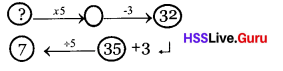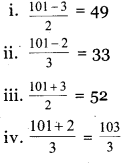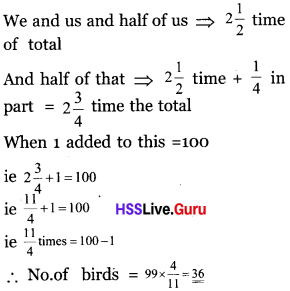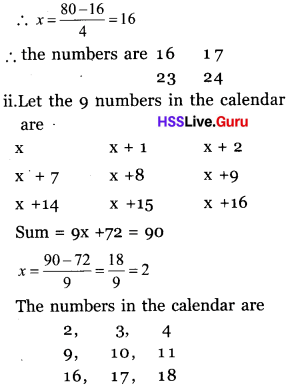# Kerala Syllabus 8th Standard Maths Solutions Chapter 2 Equations

## Kerala State Syllabus 8th Standard Maths Solutions Chapter 2 Equations

### Equations Text Book Questions and Answers

Textbook Page No. 34

Kerala Syllabus 8th Standard Maths Solution Chapter 2 Question 1.
“Six more marks and I would’ve got full hundred marks in the maths test.” Rajan was sad. How much mark did he actually get? the num¬ber.
Solution:
The marks Rajan got is six less from 100
∴ 100 – 6 = 94

Kerala Syllabus Class 8 Maths Solutions Chapter 2 Question 2.
Mother gave Rs.6o to Lissy for buying hooks. She gave back the 13 rupees left. For how much money did she buy books?
Solution:
Money Lissy got from mother = Rs. 6o
Amount she returned = Rs. 13
Amount Lissy used to buy books = 60 – 13 = Rs. 47

Kerala Syllabus 8th Standard Maths Notes Chapter 2 Question 3.
Gopalan bought a bunch of bananas. 7 of them were rotten which he threw away. Now there are 46. How many bananas were there in the bench?
Solution:
No. of rotten bananas = 7
No. of bananas left =46
∴ Total number of bananas
= 46 + 7 = 53

Hss Live Guru 8th Maths Chapter 2  Question 4.
Vimala spent 163 rupees shopping and now she has 217 rupees. How much money did she have at first?
Solution:
Amount spent by Vimala for shopping = Rs. 163
Amount left with her after shopping Rs. 217
Total amount = 163 + 217 = Rs. 380

Hsslive Guru 8th Class Maths Chapter 2 Question 5.
264 added to a number makes it 452. What is the number?
Solution:
Number = 452 – 264 = 188

Kerala Syllabus 8th Standard Notes Maths Chapter 2 Question 6.
198 subtracted from a number makes it 163. What is the number.
Solution:
Number = 198 + 163 = 361

Textbook Page No. 35

8th Scert Maths Solutions Chapter 2 Question 1.
In a company the manager’s salary in five times that of a peon. The manager gets Rs, 40,000 a month. How much does a peon get a month?
Solution:
Salary of the manager = Rs.40,000.
$$\frac{1}{5}$$ th salary of the manager in the salary of the peon.
∴ Salary of the peon = $$\frac{40000}{5}$$
= 8000

Kerala Syllabus 8th Standard Maths Textbook Solutions Question 2.
The travellers of a picnic split equally the 5200 rupees spent. Each gave 1300 rupees. How many travellers were there?
Solution:
Total amount spent = Rs. 5200
Share of one = Rs.1300
∴ Members in the group = $$\frac{5200}{1300}$$ = 4

Kerala Syllabus 8th Maths Solutions Chapter 2 Question 3.
A number multiplied by 12 gives 756. What is the number?
Solution:
Number = $$\frac{756}{12}$$ = 63

Class 8 Maths Chapter 2 Kerala Syllabus Question 4.
A number divided by 21 gives 756. What is the number
Solution:
Number 756 × 21 = 15,876

Textbook Page No. 37

8th Standard Maths Guide Kerala Syllabus Chapter 2 Question 1.
Anita and her friends bought pens. For five pens bought toge¬ther, they got a discount of three rupees and it cost them 32 rupees. Had they bought the pens separately, how much would each have to spend?
Solution:
Cost for 5 pens = Rs. 32
discount = Rs.3
Real cost = 32 + 3 = 35
∴ Real cost of 1 pen = $$\frac{35}{5}$$ = 78th Class Maths Notes Kerala Syllabus Chapter 2 Question 2.
The perimeter of a rectangle is 25 metres and one of its side is 5m. How many metres is the other side?
Solution:
Perimeter of the rectangle = 25m
One side = 5m
Double of the sum of length and breadth is perimeter = $$\frac{25}{2}$$ – 5 = 7.5Maths Class 8 Kerala Syllabus Chapter 2 Question 3.
In each of the problems below, the result of doing some operations on a number is given. Find the number?
(i) Three added to double is 101
(ii) Two added to triple is 101
(iii) Three substracted from double is 101
(iv) Two substracted from triple is 101
Solution:Hsslive 8th Class Maths Chapter 2 Question 4.
Half a number added to the number gived III. What is the number?
Solution:
1$$\frac{1}{2}$$ of the numbers = 111
ie $$\frac{3}{2}$$ of the number = 111
number = 111 × $$\frac{2}{3}$$ = 74

Hss Live Guru 8 Maths Chapter 2 Question 5.
A piece of folk maths. A child asked a flock of birds, ‘How many are you”? A bird replied
“We and us again
with half of us
And half of that
with one more.
would make hundred” How many birds were there?
Solution:
We and us ⇒ double (2 times)Textbook Page No. 41

Hsslive Guru Maths 8th Standard Chapter 2 Question 1.
The perimeter of a rectangle is 80 metre and its length is one metre more than twice the breadth. What are its length and breadth?
Solution:
If x be the breadth, Then length
= 2x + 1
2 (x + 2x + 1) = 80
2(3x + 1) = 80
6x + 2 = 80
6x = 80 – 2
x = $$\frac{80-2}{6}$$ = 13.
Length = 2 × 13 + 1 = 27 metre

Question 2.
From a point on a line another line is to be drawn such that the angle on one side is 50° more than the angle on the other side. How much is the smaller angle?
Solution:
Let one angle is x, the second angle = x + 50
Sum of two angles on a line = 180°
ie x + x + 50 = 180°
2x + 50 = 180°, 2x = 180 – 50
x = $$\frac{180-50}{2}$$ = 65
The angles are 65°, 115°

Question 3.
The price of a book is 4 rupees more than the price of a pen. The price of a pencil is 2 rupees less than the price of the pen. The total price of 5 books, 2 pens and 3 pencils is 74 rupees. What is the price of each?
Solution:
Let the cost of a pen = x
cost of a book = x + 4
Cost of a pencil = x – 2
ie.5(x + 4) + 2x + 3(x – 2) = 74
5x + 20 + 2x + 3x – 6 = 74
10 x + 14 = 74, 10x = 74 – 14
x = $$\frac{74-14}{10}$$ = 6
Cost of pen = Rs. 6
Cost of book = Rs. 10
Cost of pencil = Rs. 4

Question 4.
(i) The sum of three consecutive natural numbers is 36. What are the numbers
(ii) The sum of three consecutive even numbes is 36. What are the numbers?
(iii) Can the sum of three consecutive odd numbers be 36. Why?
(iv) The sum of three consecutive odd numbers is What are the numbers?
(v) The sum of three consecutive natural numbers is 33. What are the numbers?
Solution:
x + x + 1 + x + 2 = 36
3x + 3 = 36
x = $$\frac{36-3}{3}$$ = 11
∴ Numbers are 11, 12, 13
x – 1 + x + x + 1 = 36
3x = 36
x = 12
Or
∴ Numbers are 11, 12, 13

(ii) (x – 2) + x + (x + 2) = 36
3x = 36
x = 12
∴ The numbers are 10, 12, 14

(iii) The sum of three odd numbers is odd.
∴ It is not possible to get 36 as the sum of three odd numbers.

(iv) (x – 2) + x + (x + 2) = 33
3x = 33
x = 11
∴ Numbers are 9, 11, 13

(v) (x – 1)+ x + (x + 1) = 33
3x = 33
x = 11
The numbers are 10, 11, 12

Question 5.
(i) In a calender, a square of four numbers is marked. The sum of the numbers is 80. What are the numbers?
(ii) A square of nine numbers is marked in a calendar. The sum of all there numbers is 90. What are the numbers?
Solution:
(i) Let,
x x + 1
x + 7 x + 8
four numbers in the square of 4 numbers.
Sum of the numbers = x + (x + 1) + (x + 7) + (x + 8) = 80
4x + 16 = 80, 4x = 80 – 16Textbook Page No. 44

Question 1.
Ticket rate for the science exhibition is rupees 10 for a child and 25 rupees for the adult. Rs. 740 was got from 50 persons. How many children among them?
Solution:
Let the numbers of children be x
Then the number of adult = 50 – x
∴ 10 x + 25 (50 – x) = 740
10 x + 1250 – 25 x = 740
1250 – 15 x = 740
15 x = 1250 – 740
x = $$\frac{1250-740}{15}$$ = 34
Number of adults = 50 – 34 = 16

Question 2.
A class has the same numbers of girls and boys. Only 8 boys were absent on a particular day and then the number of girls was double the number of boys and girls?
Solution:
Let the number of boys =number of girls = x
2 (x – 8) = x
2x – 16 = x, 2x – x = 16
x – 16 = 0
∴ x = 16
∴ The number of boys = No. of girls = 16

Question 3.
Ajayan is ten years older than Vijayan. Next year Ajayan’s age would be double that of Vijayan. What are their ages now?
Solution:
Let the age of Vijayan = x
Age of Ajayan = x + 10
Age of Vijayan after an year = x + 1
Age of Ajayan after an year = x + 11
2 (x + 1) = x + 11
2x + 2 = x + 11
2x – x = 11 – 2
x = 9
∴ Age of Vijayan = 9
Age of Ajayan = 19

Question 4.
Five times a number is equal to three times the sum of the number and 4. What is the number?
Solution:
Let the number = x
Five times number = 5x
4 more than the number = x + 4
Three times of it = 3(x + 4)
ie. 5 x = 3 (x + 4)
5 x = 3x + 12
5 x – 3 x = 12
2 x = 12
x = 6

Question 5.
In a co-operative society, the number of men is thrice the number of women 29 women and 16 men more joined the society and now the number of men is double the number of women. How many women were there in the society at first?
Solution:
Let the number of women = x
No of men = 3 x
No. of women when 29 more were joined = x + 29
No. of men when 16 more were joined = 3x + 16
3 x + 16 = 2 (x + 29)
3x + 16 = 2x + 58
3x – 2x = 58 – 16
x = 42
No. of women = 42;
No. of men = 3 × 42 = 126

Question 1.
“If you give rupees 5 to me both of us have equal amounts with us”. Ajay told to Vineeth. Then Vineeth told to Ajay “ If you give rupees 5 to me I will have 5 times more money than yours. Find out the amount both of them have?
Solution:
Let Ajay has Rs.x with him and when Vineeth gives Rs. 5, both of them have x + 5 rupees with them. If Vineeth gets RS.5 he has 5 times more than Ajay.
x + 5 + 5 = 5x
4 x = 10
x = $$\frac{10}{4}=\frac{5}{2}$$ = 2.5 Rupees
Amount with Ajay = Rs. 2.50
Amount with Vineeth = Rs.12.50

Question 2.
The perimeter of a triangle is 49 cm. One side is 7 cm more than the second side and 5 cm less than the third side. Find out the lengths of three sides?
Solution:
Let the second side = xcm
First side = x + 7 cm
Third side = x + 12 cm
Perimeter = x + x + 7 + x + 12 = 49
= 3x = 49 – 19 = 30
3x = 20, x = 10 cm
The sides are = 10 cm, 17 cm, 22 cm

Question 3.
The sum of two numbers is 9, 8 times the smaller number is 2 more than 6 times the bigger number. Write an equation to find the numbers?
Solution:
Let the smaller number = x
Bigger number = 9 – x
Equation is, 8 x = 6 (9 – x) + 2

Question 4.
Anitha and friends bought pens. When they bought a packet of 5 pens they got a discount of rupees 2. They paid rupees 18. Find the cost of each one buys separately?
Solution:
Total cost in including discount = Rs. 20
Cost of 5 pens = Rs.20
Cost of 1 pen = $$\frac{20}{5}$$ = 4

Question 5.
The cost of a chair and a table is Rs. 1500. The cost of table is 4 times the cost of chair. Find the expense of each?
Solution:
Let the cost of chair = x
Cost of table = 4x
x + 4 x = 1500
5x = 1500
x = 300
cost of chair = Rs. 300
Cost of table = Rs. 1200

Question 6.
There were 25 questions in the examination written by Jafar. Each correct answer gets 2 marks. There is a loss of mark for each wrong answer. Jafar answered all the questions. He got 35 marks. Find^ut the number of correct answers?
Solution:
Let the number of correct answers = x
Total number of questions = 25
No. of wrong answers = 25 – x
Marks for correct answers = 2x
marks losses for wrong answers = 25
ie. 2x – (25 – x) = 25
2x – 25 + x = 35
3x = 60
x = $$\frac{60}{3}$$ = 20, ∴ number of correct answers = 20.